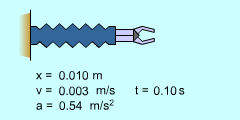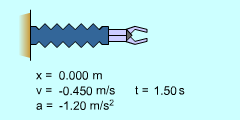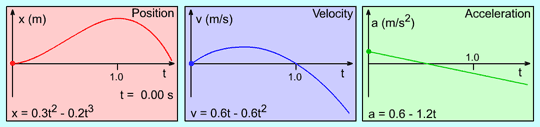Ch 1. Particle General Motion Multimedia Engineering Dynamics Position,Vel & Accel. Accel. varyw/ Time Accel. Constant Rect. Coordinates Norm/Tang. Coordinates Polar Coordinates RelativeMotion
 Chapter - Particle - 1. General Motion 2. Force & Accel. 3. Energy 4. Momentum - Rigid Body - 5. General Motion 6. Force & Accel. 7. Energy 8. Momentum 9. 3-D Motion 10. Vibrations Appendix Basic Math Units Basic Equations Sections Search eBooks Dynamics Fluids Math Mechanics Statics Thermodynamics Author(s): Kurt Gramoll ©Kurt GramollDYNAMICS - CASE STUDY SOLUTION Solution of a)Arm motion from 0 ≤ t ≤ 1 seconds Take the time derivative of the position to get an equation for the velocity as a function of time:      v(t) = dx(t)/dt = d(0.3 t2 - 0.2 t3)/dt      v(t) = 0.6 t - 0.6 t2 m/sArm motion from 1≤ t ≤ 1.5 seconds Solution of b) Take the time derivative of the velocity to get an equation for the acceleration as a function of time:      a(t) = dv(t)/dt = d(0.6 t - 0.6 t2 )/dt      a(t) = 0.6 - 1.2 t m/s2Position-Velocity-Acceleration Curve Relationships

Practice Homework and Test problems now available in the 'Eng Dynamics' mobile app
Includes over 400 problems with complete detailed solutions.
Available now at the Google Play Store and Apple App Store.